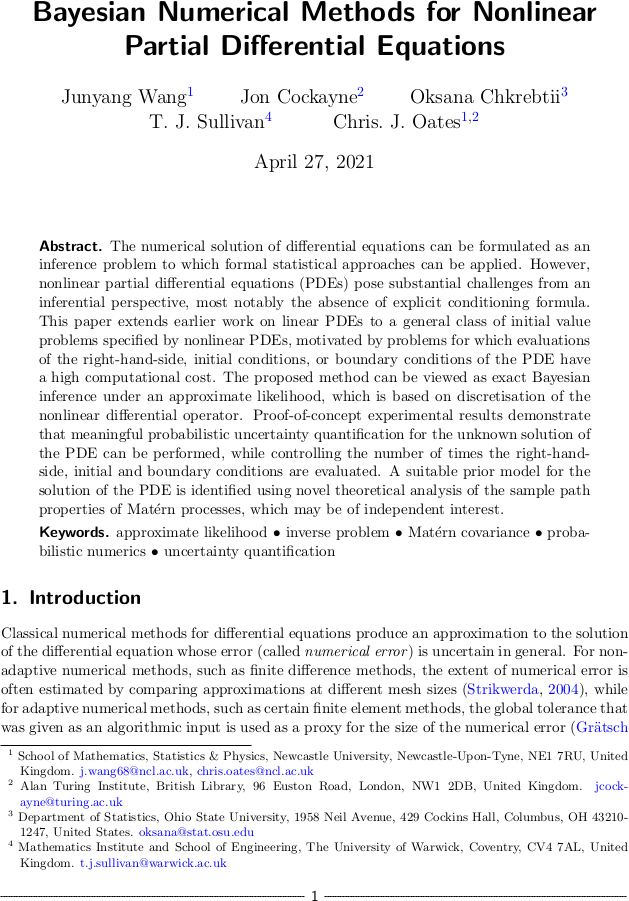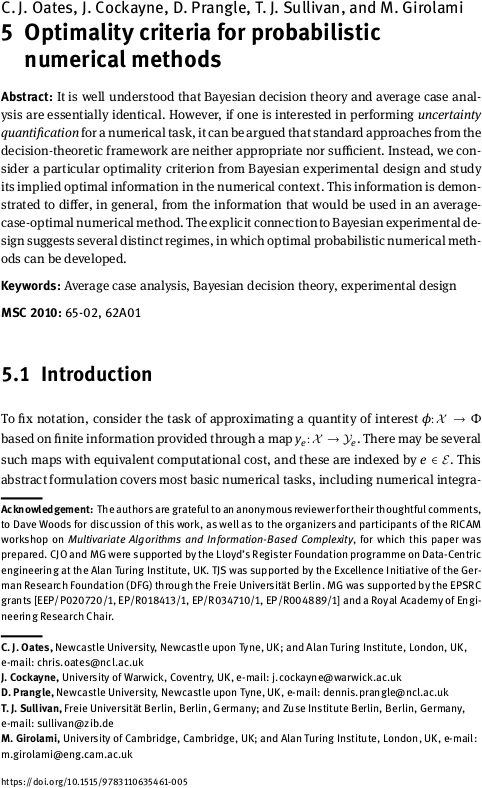# Tim Sullivan

### #cockayne

Clear Search### Bayesian numerical methods for nonlinear PDEs

Junyang Wang, Jon Cockayne, Oksana Chkrebtii, Chris Oates, and I have just uploaded a preprint of our recent work “Bayesian numerical methods for nonlinear partial differential equations” to the arXiv. This paper continues our study of (approximate) Bayesian probabilistic numerical methods (Cockayne et al., 2019), in this case for the challenging setting of nonlinear PDEs, with the goal of realising a posterior distribution over the solution of the PDE that carries a meaningful expression of uncertainty about the solution's true value given the discretisation error that has been incurred.

Abstract. The numerical solution of differential equations can be formulated as an inference problem to which formal statistical approaches can be applied. However, nonlinear partial differential equations (PDEs) pose substantial challenges from an inferential perspective, most notably the absence of explicit conditioning formula. This paper extends earlier work on linear PDEs to a general class of initial value problems specified by nonlinear PDEs, motivated by problems for which evaluations of the right-hand-side, initial conditions, or boundary conditions of the PDE have a high computational cost. The proposed method can be viewed as exact Bayesian inference under an approximate likelihood, which is based on discretisation of the nonlinear differential operator. Proof-of-concept experimental results demonstrate that meaningful probabilistic uncertainty quantification for the unknown solution of the PDE can be performed, while controlling the number of times the right-hand-side, initial and boundary conditions are evaluated. A suitable prior model for the solution of the PDE is identified using novel theoretical analysis of the sample path properties of Matérn processes, which may be of independent interest.

Published on Tuesday 27 April 2021 at 10:00 UTC #preprint #prob-num #wang #cockayne #chkrebtii #oates### Optimality of probabilistic numerical methods

The paper “Optimality criteria for probabilistic numerical methods” by Chris Oates, Jon Cockayne, Dennis Prangle, Mark Girolami, and myself has just appeared in print:

C. J. Oates, J. Cockayne, D. Prangle, T. J. Sullivan, and M. Girolami. “Optimality criteria for probabilistic numerical methods” in Multivariate Algorithms and Information-Based Complexity, ed. F. J. Hickernell and P. Kritzer. Radon Series on Computational and Applied Mathematics 27:65–88, 2020. doi:10.1515/9783110635461-005

Abstract. It is well understood that Bayesian decision theory and average case analysis are essentially identical. However, if one is interested in performing uncertainty quantification for a numerical task, it can be argued that standard approaches from the decision-theoretic framework are neither appropriate nor sufficient. Instead, we consider a particular optimality criterion from Bayesian experimental design and study its implied optimal information in the numerical context. This information is demonstrated to differ, in general, from the information that would be used in an average-case-optimal numerical method. The explicit connection to Bayesian experimental design suggests several distinct regimes, in which optimal probabilistic numerical methods can be developed.

Published on Sunday 31 May 2020 at 08:00 UTC #publication #prob-num #oates #cockayne #prangle #girolami### PhD Project on Adaptive Probabilistic Meshless Methods

There is an opening for a PhD student to work with me and co-PIs Jon Cockayne and James Kermode on the project “Adaptive probabilistic meshless methods for evolutionary systems” as part of the EPSRC Centre for Doctoral Training in Modelling of Heterogeneous Systems at the University of Warwick.

This project will develop and implement a new class of numerical solvers for evolving systems such as interacting fluid-structure flows. To cope with extreme strain rates and large deformations these new solvers will be adaptive and meshless, and they will also implicitly represent their own solution uncertainty, thus enabling optimal design and uncertainty quantification. This exciting project brings together aspects of continuum mechanics, numerical methods for partial differential equations, and statistical machine learning.

Interested students should contact me and the other PIs with informal queries. Formal applications should use the HetSys application page.

Published on Monday 20 April 2020 at 08:00 UTC #group #job #phd #warwick #hetsys #kermode #cockayne### Bayesian probabilistic numerical methods in SIAM Review

The 2019 Q4 issue of SIAM Review will carry an article by Jon Cockayne, Chris Oates, Mark Girolami, and myself on the Bayesian formulation of probabilistic numerical methods, i.e. the interpretation of deterministic numerical tasks such as quadrature and the solution of ordinary and partial differential equations as (Bayesian) statistical inference tasks.

J. Cockayne, C. J. Oates, T. J. Sullivan, and M. Girolami. “Bayesian probabilistic numerical methods.” SIAM Review 61(4):756–789, 2019. doi:10.1137/17M1139357

Abstract. Over forty years ago average-case error was proposed in the applied mathematics literature as an alternative criterion with which to assess numerical methods. In contrast to worst-case error, this criterion relies on the construction of a probability measure over candidate numerical tasks, and numerical methods are assessed based on their average performance over those tasks with respect to the measure. This paper goes further and establishes Bayesian probabilistic numerical methods as solutions to certain inverse problems based upon the numerical task within the Bayesian framework. This allows us to establish general conditions under which Bayesian probabilistic numerical methods are well defined, encompassing both the nonlinear and non-Gaussian contexts. For general computation, a numerical approximation scheme is proposed and its asymptotic convergence established. The theoretical development is extended to pipelines of computation, wherein probabilistic numerical methods are composed to solve more challenging numerical tasks. The contribution highlights an important research frontier at the interface of numerical analysis and uncertainty quantification, and a challenging industrial application is presented.

Published on Thursday 7 November 2019 at 07:00 UTC #publication #bayesian #siam-review #prob-num #cockayne #girolami #oates### Optimality of probabilistic numerical methods

Chris Oates, Jon Cockayne, Dennis Prangle, Mark Girolami, and I have just uploaded a preprint of our paper “Optimality criteria for probabilistic numerical methods” to the arXiv.

Abstract. It is well understood that Bayesian decision theory and average case analysis are essentially identical. However, if one is interested in performing uncertainty quantification for a numerical task, it can be argued that the decision-theoretic framework is neither appropriate nor sufficient. To this end, we consider an alternative optimality criterion from Bayesian experimental design and study its implied optimal information in the numerical context. This information is demonstrated to differ, in general, from the information that would be used in an average-case-optimal numerical method. The explicit connection to Bayesian experimental design suggests several distinct regimes in which optimal probabilistic numerical methods can be developed.

Published on Tuesday 15 January 2019 at 11:00 UTC #preprint #prob-num #oates #cockayne #prangle #girolami